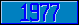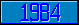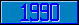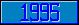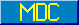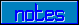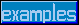## \$%MTXTRP

### M[UMPS] by Example

```
2-Jul–96, 6:54:35

Routine Save for Matrix Mathematics Library function \$%MTXTRP^MATH

;

; Unless otherwise noted, the code below

; was approved in document X11/96–26

;

; If corrections have been applied,

; first the original line appears,

; with three semicolons at the beginning of the line.

;

; Then the source of the correction is acknowledged,

; then the corrected line appears, followed by a

; line containing three semicolons.

;

MTXTRP(A,R,M,N) ;

; Transpose A[M,N], result goes to R[N,M]

IF \$DATA(A)<10 QUIT 0

IF \$GET(M)<1 QUIT 0

IF \$GET(N)<1 QUIT 0

;

NEW I,J,K,D1,V1,D2,V2

FOR I=1:1:M+N–1 FOR J=1:1:I+1\2 DO

. SET K=I-J+1

. IF K=J DO  QUIT

. . SET V1=\$GET(A(J,J)),D1=\$DATA(A(J,J))#2

. . IF J'>N,J'>M KVALUE R(J,J) SET:D1 R(J,J)=V1

. . QUIT

. ;

. SET V1=\$GET(A(K,J)),D1=\$DATA(A(K,J))#2

. SET V2=\$GET(A(J,K)),D2=\$DATA(A(J,K))#2

. IF K'>M,J'>N KVALUE R(K,J) SET:D2 R(K,J)=V2

. IF J'>M,K'>N KVALUE R(J,K) SET:D1 R(J,K)=V1

. QUIT

QUIT 1

;===

;

;

;

```